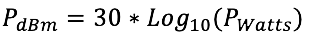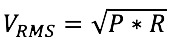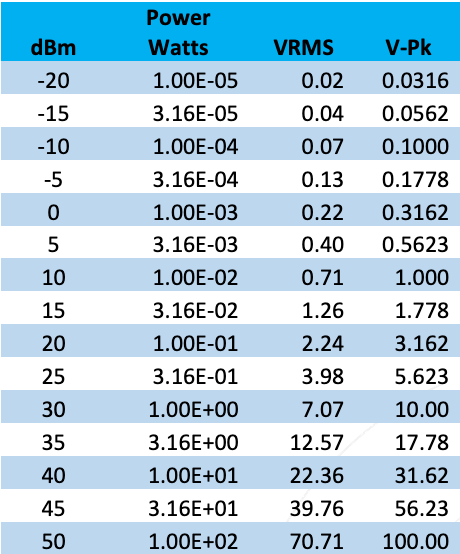|
|### What are dBm?

March 22, 2023

RF signal power is often expressed in a logarithmic format. dBm refers to the ratio of the RF power to 1 milliwatt in logarithmic format. For instance, 1 Watt is 1000 milliwatts, so the ratio is 1000 and Log10(1000) gives 30 dBm. In general:The RMS voltage of an RF signal in a characteristic impedance R is given by:Some RMS and peak voltages in a 50Ω system are shown in the table below.Table – RMS and Pk RF Voltage vs dBm

Notably, the peak RF voltages at +10 dBm and +30 dBm are 1V and 10V respectively. This is useful to know when evaluating low frequency signal levels with an oscilloscope.

See this and more important radio frequency charts and formulas in this comprehensive application note